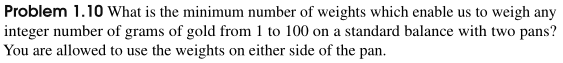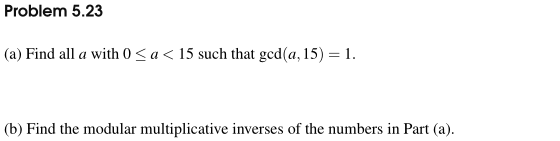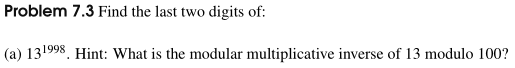Math Challenge II-B Textbooks

Please see the sections below for answer keys, topics covered, and sample problems for each of the MC II-B textbooks.

Algebra

The answer key for MC II-B Algebra is available by clicking here.

Chapter Topics

1. Basic Factoring
3. Complex Numbers
4. Complex Numbers
5. Polynomials
7. Solving Equations with Fractions and Absolute Values
9. Exponents/Logarithms
10. Math Contest Review

Sample Questions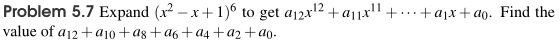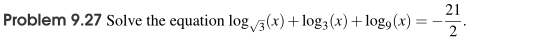Geometry

The answer key for MC II-B Geometry is available by clicking here.

Chapter Topics

1. Special Angles
2. Special Angles II
3. Trigonometry
4. Trigonometry II
5. Areas
6. Areas II
7. Circles
8. Circles II
9. Solid Geometry
10. Math Contest Review

Sample Questions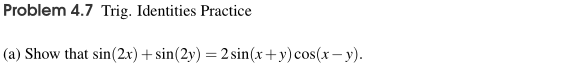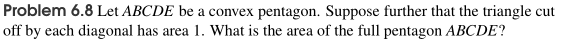Combinatorics

The answer key for MC II-B Combinatorics is available by clicking here.

Chapter Topics

1. Counting Fundamentals
2. Grouping and Spacing
3. Stars and Bars
4. Sets and PIE
6. Identities
7. Recurrences
8. Probability Fundamentals
10. Math Contest Review

Sample Questions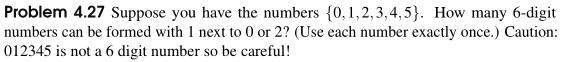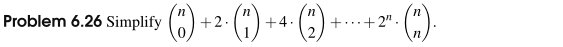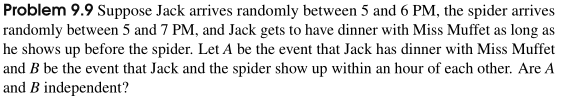Number Theory

The answer key for MC II-B Number Theory is available by clicking here.

Chapter Topics

1. Place Values and Number Bases
2. Divisibility, Divisors, and Multiples
3. Problem Solving with Primes
4. Modular Arithmetic
5. Problem Solving with Modular Arithmetic
6. Fundamental Theorems in Modular Arithmetic
7. Advanced Theorems in Modular Arithmetic
8. Diophantine Equations
9. Solving Equations with the Floor Function
10. Math Contest Review

Sample Questions